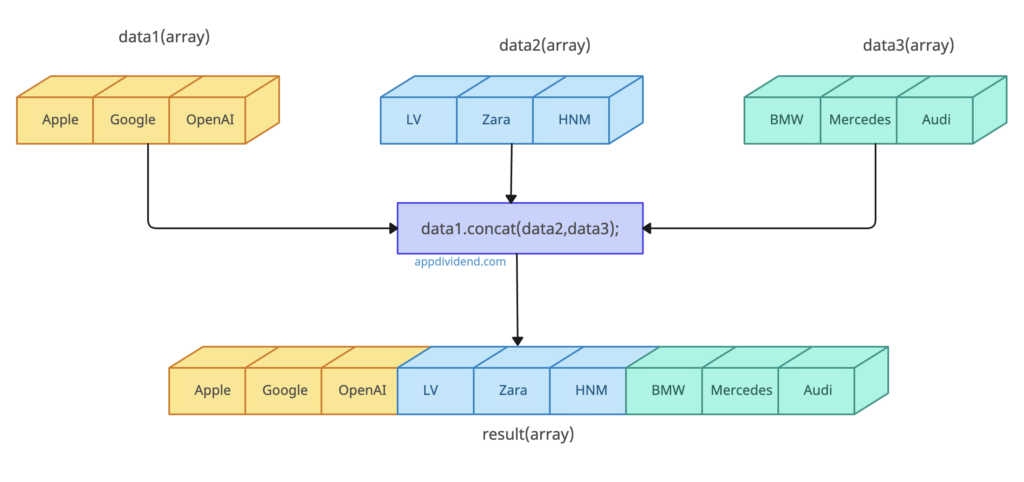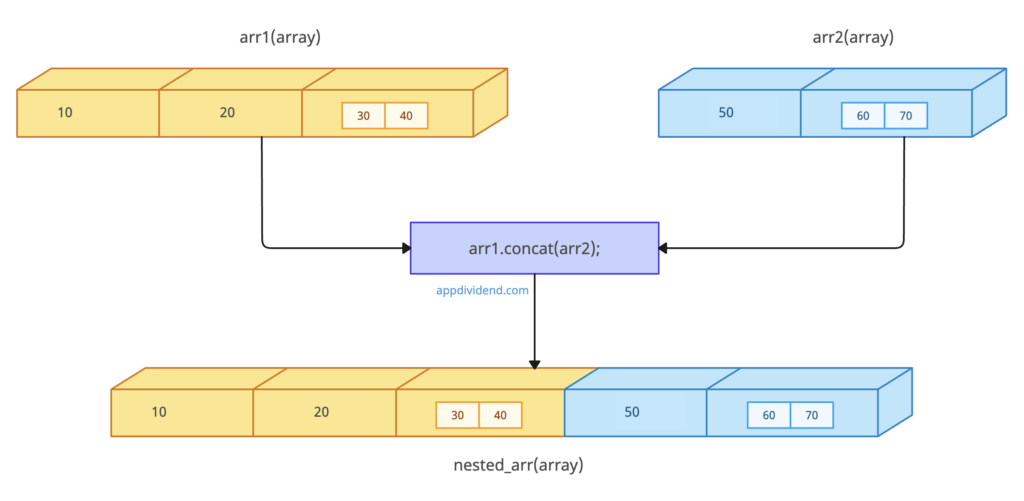# JavaScript Array concat() Method

JavaScript Array concat() method is used to merge two or more arrays.

It does not change the existing array.

## Syntax

``array1.concat(array2, array3, ..., arrayX)``

## Parameters

array1(required): The array to which other arrays will be concatenated.

## Return value

Returns a new array which is the content from the joined arrays.

## Example 1: How to Use Array concat() Method

``````let data1 = ['Apple', 'Google', 'OpenAI'];
let data2 = ['LV', 'Zara', 'HNM'];
let result = data1.concat(data2);
console.log(result);``````

Output

``[ 'Apple', 'Google', 'OpenAI', 'LV', 'Zara', 'HNM' ]``

## Example 2: Concatenating three Arrays``````let data1 = ['Apple', 'Google', 'OpenAI'];
let data2 = ['LV', 'Zara','HNM'];
let data3 = ['BMW', 'Mercedes', 'Audi'];
let result = data1.concat(data2,data3);
console.log(result);``````

Output

``[ 'Apple', 'Google', 'OpenAI', 'LV', 'Zara', 'HNM', 'Mercedes', 'Audi' ]``

## Example 3: Concatenating nested arrays``````let arr1 = [10, 20, [30, 40]];
let arr2 = [50, [60, 70]];

let nested_arr = arr1.concat(arr2);
console.log(result);``````

Output

``[ 10, 20, [ 30, 40 ], 50, [ 60, 70 ] ]``

## Example 4: Using sparse arrays

``````console.log([10, , 30].concat([50, 60]));

console.log([10, 20].concat([50, , 70]));
``````

Output

``````[ 10, <1 empty item>, 30, 50, 60 ]
[ 10, 20, 50, <1 empty item>, 70 ]
``````

## Example 5: Calling concat() on non-array objects

``````console.log(Array.prototype.concat.call({}, 20, 30, 40));

console.log(Array.prototype.concat.call(1, 2, 3));
``````

Output

``````[ {}, 11, 21, 19 ]
[ [Number: 1], 2, 3 ]``````

## Browser Compatibility

1. Google Chrome 1 and above
2. Edge 12 and above
3. Firefox 1 and above
4. Opera 4 and above
5. Safari 1 and above

This site uses Akismet to reduce spam. Learn how your comment data is processed.